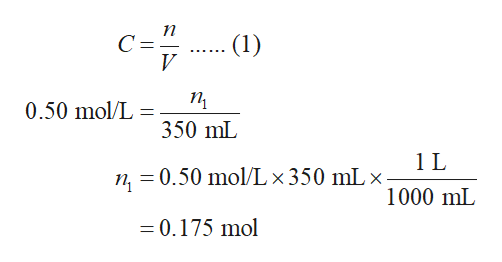# Suppose 26.8g of sodium bromide is dissolved in 350.mL of a 0.50 M aqueous solution of silver nitrate. Calculate the final molarity of bromide anion in the solution. You can assume the volume of the solution doesn't change when the sodium bromide is dissolved in it.Be sure your answer has the correct number of significant digits.

Question
76 views

Suppose

26.8g

of sodium bromide is dissolved in

350.mL

of a

0.50 M

aqueous solution of silver nitrate.

Calculate the final molarity of bromide anion in the solution. You can assume the volume of the solution doesn't change when the sodium bromide is dissolved in it.

check_circle

Step 1

The number of moles AgNO3 in 0.50 M 350 mL solution (n1) is calculated using equation (1) in which n is the number of moles, V is the volume of solution, and C is the molarity of the solution. The number of moles of AgNO3 (n1) is calculated as follows:help_outlineImage Transcriptioncloseп C= (1) ...... п 0.50 mol/L 350 mL n, = 0.50 mol/L × 350 mL × 1000 mL = 0.175 mol fullscreen
Step 2

The number of moles of NaBr (n2) is calculated using equation (2) in which n is the number of moles, m is the mass of a substance, and M is the molar mass of substance.

Step 3

0.175 mol of AgBr produces 0.175 mol (n1) of silver ion (Ag+) in the solution. 0.260 mol of NaBr produces 0.260 mol (n2) of bromide ion (Br-) in the solution. One mole of silver ion reacts with one mole of bromide ion to produce one mole of silver b...

### Want to see the full answer?

See Solution

#### Want to see this answer and more?

Solutions are written by subject experts who are available 24/7. Questions are typically answered within 1 hour.*

See Solution
*Response times may vary by subject and question.
Tagged in

### General Chemistry